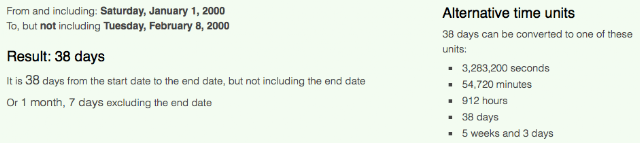## Sunday, December 20, 2015

### 33 34 38 39 96 144 | The Death of Derrick Thomas, At Age 33, 9-Days After Super Bowl 34

Derrick = 4+5+9+9+9+3+2 = 41
Vincent = 4+9+5+3+5+5+2 = 33
Thomas = 2+8+6+4+1+1 = 22/31
Derrick Vincent Thomas = 96/105
Derrick Thomas = 63/72

Derrick = 4+5+18+18+9+3+11 = 68
Vincent = 22+9+14+3+5+14+20 = 87
Thomas = 20+8+15+13+1+19 = 76
Derrick Vincent Thomas = 231
Derrick Thomas = 144

DT = 42 (D = 4; T = 20 (2))
Missouri = 4+9+1+1+6+3+9+9 = 42
February = 6+5+2+9+3+1+9+7 = 42

1/1/1967 = 1+1+19+67 = 88
1/1/1967 = 1+1+1+9+6+7 = 25
1/1/67 = 1+1+67 = 69

2/8/2000 = 2+8+20+00 = 30
2/8/2000 = 2+8+2+0+0+0 = 12
2/8/00 = 2+8+00 = 10Notice he wore #58Freemasonry = 58
Derrick Thomas died exactly 38-days after his 33rd birthday.

Death = 4+5+1+20+8 = 38

If you count the end date, he died 39-days after his birthday, on February 8, the 39th day of the year.  He died 9-days after the St. Louis Rams won the Super Bowl, 23-16, with a sum of 39 points, and also being from the state of Missouri, like the Kansas City Chiefs.  Again, Thomas died in Missouri.Counting the end date, it was a span of 39-days

Notice his death involved a car accident, with his car crashing off of the road under suspicious circumstances.  His death ended his career after 11 NFL Seasons.  He died 9-days after Super Bowl 34.  Keep in mind, 34 is the 9th Fibonacci Number, and '34' is a number connected to murder.

Murder = 4+3+9+4+5+9 = 34